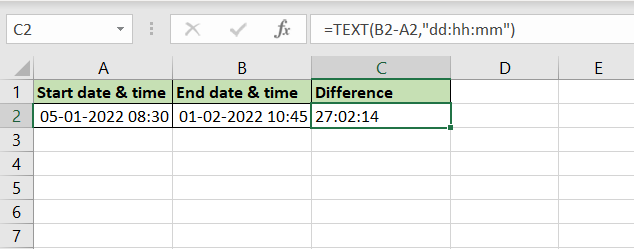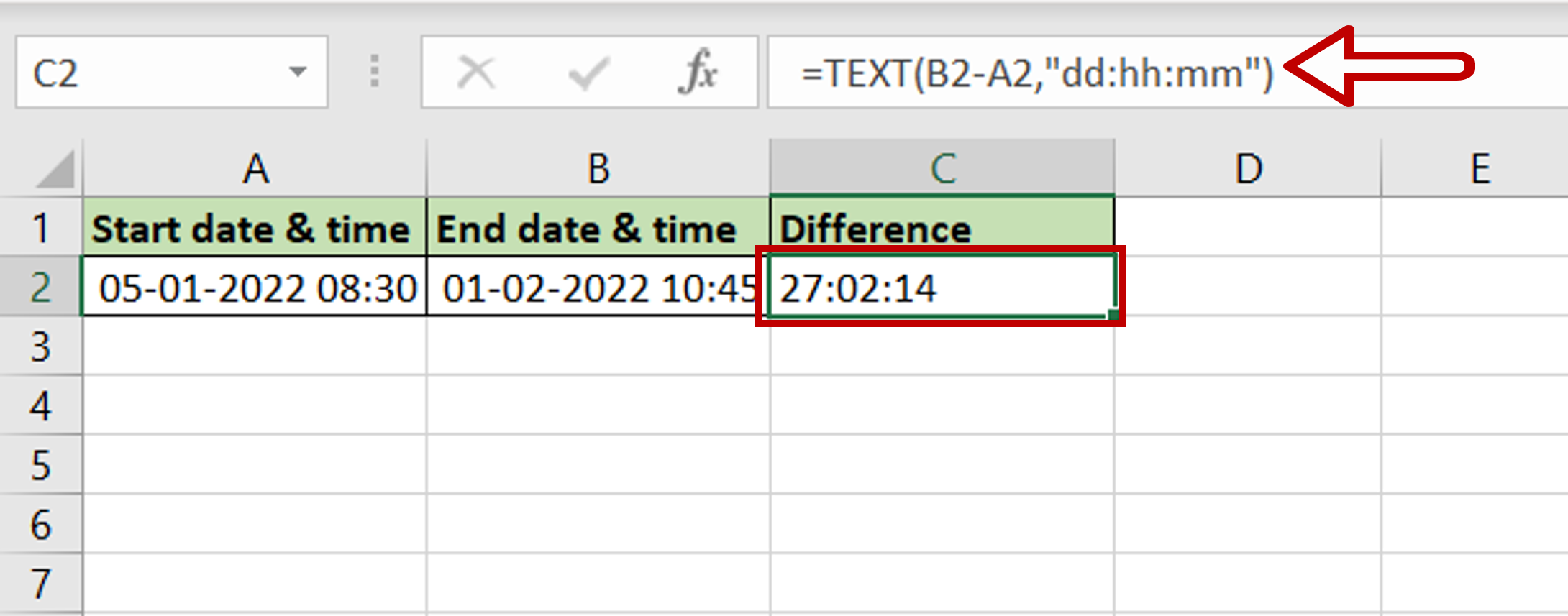# How to calculate date and time difference in Excel

You can watch a video tutorial here.Excel treats date and time formats differently from numbers and text. Because of the different scales i.e. 24 hours, 60 minutes, and 60 seconds, when adding minutes and seconds they cannot be treated like regular numbers. In Excel, to be able to add or subtract dates and times, you need to format them as dates and times for the operation to work correctly. Assume you have employed a consultant who charges you a daily and an hourly fee. You need to compute the total days and hours worked by the consultant to arrive at the total fee to be paid. To do this, you will need to find the difference between the dates and the times to get the total number of days and hours worked. When you subtract the dates, the result is returned as the number of days. To get the number of hours and days displayed as such, we use the TEXT() function.

1. TEXT() function: this applies a customized format to a number
a. Syntax: TEXT(value, format)
i. value: the number to be formatted
ii. format: the customized format

In this example, we will use the format of “dd:hh:mm” to display the days, hours, and minutes.

### Step 1 – Type the formula– Select the cell where the result is to appear
– Type the formula using cell references:
=TEXT(End date & time – Start date & time,”dd:hh:mm”)

### Step 2 – Check the result– The number of elapsed days, hours and minutes are displayed